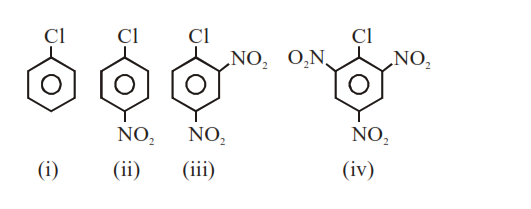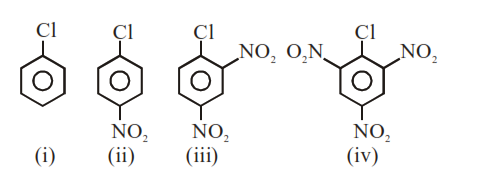# The correct order of the following compounds showing increasing tendency towards nucleophilic substitution reaction is :-`
Question:

The correct order of the following compounds showing increasing tendency towards nucleophilic substitution reaction is :-1. (iv) $<$ (iii) $<$ (ii) $<$ (i)

2. $(\mathrm{iv})<(\mathrm{i})<(\mathrm{ii})<(\mathrm{iii})$

3. (iv) $<$ (i) $<$ (iii) $<$ (ii)

4. $(\mathrm{i})<(\mathrm{ii})<(\mathrm{iii})<(\mathrm{iv})$

Correct Option: , 4

Solution:

For nucleophile substitution in aromatic halidesCorrect order is :

(i) $<$ (ii) $<$ (iii) $<$ (iv)

More $\mathrm{No}$. of $\mathrm{NO}_{2}$ substituted aromatic halide, increase the rate of nucleophile substitution reaction in aromatic halides.